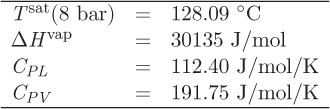+1-415-670-9189
info@expertsmind.com

# Get Solution

Determine the moles of the vapor that must be mixed
Course:- Chemical Engineering
Reference No.:- EM131156631

 TweetExpertsmind Rated 4.9 / 5 based on 47215 reviews.
Review Site
Assignment Help >> Chemical Engineering

Problem 3.30:

1 mol of liquid hexane at 10 bar, 25 °C, is mixed adiabatically and under constant pressure with hexane vapor at 180 °C. Determine the moles of the vapor that must be mixed with the liquid in order to produce a final state that consists of a vapor-liquid mixture with 75% (by mass) liquid. You may use the data given in problem 3.29.

Problem 3.31:

Methanol at 25 °C, 8 bar is heated under constant P in closed system.

a) Determine the amount of heat to produce 95% vapor

b) Determine the final state if Q = 32000 J/mol

c) Determine the final state if Q = 52000 J/mol

Additional data:Minimize

Ask Question & Get Answers from Experts
Browse some more (Chemical Engineering) Materials
 What would be the reduction in income-tax charges for the first year of operation if the sum-of-the-years-digits method were used for depreciation accounting instead of the For the following cases: How much energy is lost every day when the steam temperature is maintained at 200oC, and the surrounding average air temperature is 20.0oC, and the su Calculate the equilibrium conversion and the CSTR and PFR volumes necessary to achieve 98% of the equilibrium conversion and calculate the time to reach 90% conversion if the An effluent disposal contractor is considering entering the recycled field. At present he incinerates an organic waste containing recoverable solvents and dumps the aqueous The fictitious compound, pandemonium fluoride (PnF2) has a Ksp value in water of 3.091×10-9M3 at room temperature. Calculate the solubility of PnF2 in water. Express your answ Give the relation for strain energy in a rubber as a function of the extension ratios, and from this develop equations developing the extension ratio and the engineering stres Mention all instruments (e.g., thermometers, wattmeter, etc.) that the crew should have and identify those that they must monitor closely. Assume that all ingredients were f With this knowledge, which type of radioactive decay has the net effect of changing a neutron into a proton? Which type of decay has the net effect of turning a proton into